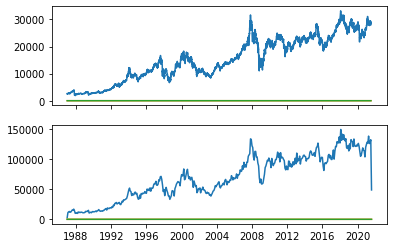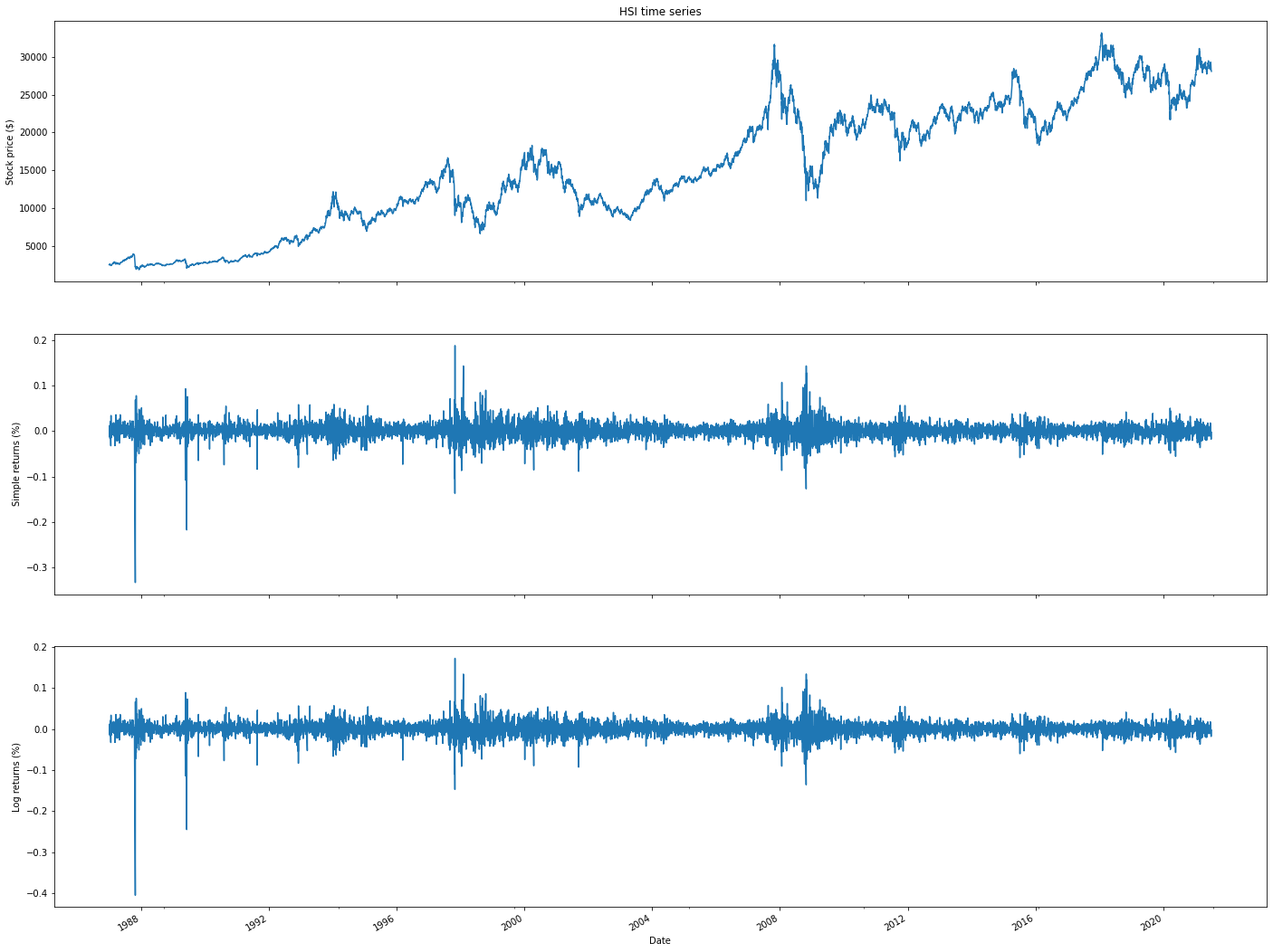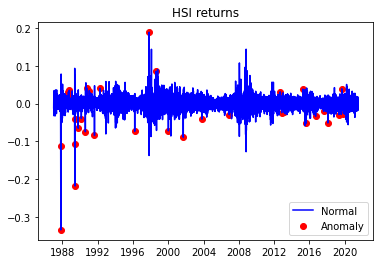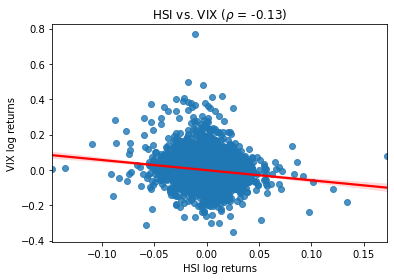# HANG SENG INDEX (^HSI) is stock market Hong Kong

## What does Hsi stand for in the stock market in Python?

HANG SENG INDEX (^HSI) is a stock market in Hong Kong.

The Hang Seng Index (HSI), often referred to as the "Hanging Seng," is a major stock market index that represents the performance of the Hong Kong stock market. It is one of the most widely followed and recognized stock indices in Asia and serves as a benchmark for the performance of Hong Kong's equity market. Here are key details about the Hang Seng Index:

Composition: The Hang Seng Index is composed of a diverse set of companies listed on the Hong Kong Stock Exchange (HKEX). It typically includes the largest and most actively traded stocks from various sectors, including finance, real estate, technology, and more. The exact composition and criteria for inclusion are periodically reviewed and adjusted by the Hang Seng Indexes Company Limited, the organization responsible for maintaining the index.

Market Capitalization Weighted: Similar to many other stock market indices, the Hang Seng Index is market capitalization-weighted. This means that the weight of each component stock in the index is determined by its market capitalization, which is the product of its stock price and the number of outstanding shares. Larger companies have a more significant impact on the index's performance.

Global Significance: While the Hang Seng Index primarily reflects the performance of Hong Kong-listed companies, it is closely watched by investors and financial professionals worldwide. It is often used as an indicator of the health of Hong Kong's economy and as a gauge of investor sentiment in the broader Asian market.

Sector Diversity: The index includes companies from various sectors, including financial services (e.g., banks and insurance companies), real estate (e.g., property developers), information technology (e.g., tech firms), consumer goods, and more. This diversity helps spread risk for investors.

Calculation: The Hang Seng Index is calculated and maintained by the Hang Seng Indexes Company Limited. It uses a formula that takes into account the market capitalization of each component stock and adjusts for events such as stock splits and mergers.

Price and Total Return Versions: Similar to other indices, there are two versions of the Hang Seng Index: the price return version and the total return version. The price return version reflects changes in the prices of the index's constituent stocks, while the total return version includes dividend income generated by those stocks.

Market Hours: The Hang Seng Index follows the trading hours of the Hong Kong Stock Exchange, typically open from 9:30 AM to 4:00 PM (local time), Monday to Friday.

Historical Performance: The Hang Seng Index's historical performance reflects the ups and downs of the Hong Kong and global economies, as well as trends in various industries.

Volatility: Like most stock indices, the Hang Seng Index can experience periods of volatility influenced by factors such as economic data, geopolitical events, interest rates, and currency fluctuations.

Investors and financial professionals use the Hang Seng Index as a reference point for assessing the performance of Hong Kong stocks, making investment decisions, and managing portfolios. It serves as a critical tool for portfolio diversification and risk management, particularly for investors with exposure to Asian markets.

Hong kong

## Why is hsi droping?

We have described below through python

!pip install yfinance
import yfinance as yf
import numpy as np
import pandas as pd
import seaborn as sns
import scipy.stats as scs
import statsmodels.api as sm
import statsmodels.tsa.api as smt
import matplotlib.pyplot as plt

#### This is the stock price of hsi from 1985 to 2021

start='1985-01-01',
end='2021-07-07',
progress=False)
df[['simple_rtn','log_rtn']].tail(20)

### Stock price of Hsi simple and log return

simple_rtnlog_rtn
Date
2021-06-07-0.004524-0.004534
2021-06-08-0.000205-0.000205
2021-06-09-0.001346-0.001347
2021-06-10-0.000130-0.000130
2021-06-110.0035930.003586
2021-06-15-0.007059-0.007084
2021-06-16-0.007043-0.007068
2021-06-170.0042810.004272
2021-06-180.0084980.008462
2021-06-21-0.010842-0.010901
2021-06-22-0.006292-0.006311
2021-06-230.0179200.017761
2021-06-240.0022690.002267
2021-06-250.0140490.013951
2021-06-28-0.000680-0.000680
2021-06-29-0.009369-0.009413
2021-06-30-0.005730-0.005747
2021-07-02-0.017952-0.018115
2021-07-05-0.005896-0.005914
2021-07-06-0.002510-0.002513

### How to get realised volatily of hsi?

def realized_volatility(x):
return np.sqrt(np.sum(x**2))
df_rv = df.groupby(pd.Grouper(freq='M')).apply(realized_volatility)
df_rv.rename(columns={'log_rtn''rv'}, inplace=True)
df_rv.rv = df_rv.rv * np.sqrt(12)
fig, ax = plt.subplots(21, sharex=True)
ax.plot(df)
ax.plot(df_rv)
[<matplotlib.lines.Line2D at 0x7f0d3d28ba10>,
<matplotlib.lines.Line2D at 0x7f0d3d2a35d0>,
<matplotlib.lines.Line2D at 0x7f0d3d2a37d0>]### How to plot realised volatility?

fig, ax = plt.subplots(31, figsize=(2420), sharex=True)
ylabel = 'Stock price ($)') df.simple_rtn.plot(ax=ax) ax.set(ylabel = 'Simple returns (%)') df.log_rtn.plot(ax=ax) ax.set(xlabel = 'Date', ylabel = 'Log returns (%)') [Text(0, 0.5, 'Log returns (%)'), Text(0.5, 0, 'Date')] ### How to plot hsi time series?import cufflinks as cf from plotly.offline import iplot, init_notebook_mode init_notebook_mode() df_rolling = df[['simple_rtn']].rolling(window=21) \ .agg(['mean''std']) df_rolling.columns = df_rolling.columns.droplevel() df_outliers = df.join(df_rolling) def indentify_outliers(rown_sigmas=3): x = row['simple_rtn'] mu = row['mean'] sigma = row['std'] if (x > mu + 3 * sigma) | (x < mu - 3 * sigma): return 1 else: return 0 df_outliers['outlier'] = df_outliers.apply(indentify_outliers, axis=1) outliers = df_outliers.loc[df_outliers['outlier'] == 1, ['simple_rtn']] fig, ax = plt.subplots() ax.plot(df_outliers.index, df_outliers.simple_rtn, color='blue', label='Normal') ax.scatter(outliers.index, outliers.simple_rtn, color='red', label='Anomaly') ax.set_title("HSI returns") ax.legend(loc='lower right') ### HSI Outlier simple return <matplotlib.legend.Legend at 0x7f0d36f254d0>r_range = np.linspace(min(df.log_rtn), max(df.log_rtn), num=1000) mu = df.log_rtn.mean() sigma = df.log_rtn.std() norm_pdf = scs.norm.pdf(r_range, loc=mu, scale=sigma) fig, ax = plt.subplots(12, figsize=(168)) # histogram sns.distplot(df.log_rtn, kde=False, norm_hist=True, ax=ax) ax.set_title('Distribution of HSI returns', fontsize=16) ax.plot(r_range, norm_pdf, 'g', lw=2, label=f'N({mu:.2f}{sigma**2:.4f})') ax.legend(loc='upper left'); /usr/local/lib/python3.7/dist-packages/seaborn/distributions.py:2557: FutureWarning: distplot is a deprecated function and will be removed in a future version. Please adapt your code to use either displot (a figure-level function with similar flexibility) or histplot (an axes-level function for histograms).  df.log_rtn.plot(title='Daily HSI returns') <matplotlib.axes._subplots.AxesSubplot at 0x7f0d3811f850> df = yf.download(['^HSI''^VIX'], start='1985-01-01', end='2021-07-07', progress=False) df.tail() df = df[['Adj Close']] df.tail() df.columns = df.columns.droplevel(0) df = df.rename(columns={'^HSI''hsi''^VIX''vix'}) df['log_rtn'] = np.log(df.hsi / df.hsi.shift(1)) df['vol_rtn'] = np.log(df.vix / df.vix.shift(1)) df.dropna(how='any', axis=0, inplace=True) corr_coeff = df.log_rtn.corr(df.vol_rtn) ax = sns.regplot(x='log_rtn', y='vol_rtn', data=df, line_kws={'color''red'}) ax.set(title=f'BVSP vs. VIX ($\\rho$= {corr_coeff:.2f})', ylabel='VIX log returns', xlabel='HSI log returns') [Text(0, 0.5, 'VIX log returns'), Text(0.5, 0, 'HSI log returns'), Text(0.5, 1.0, 'HSI vs. VIX ($\\rho\$ = -0.13)')]